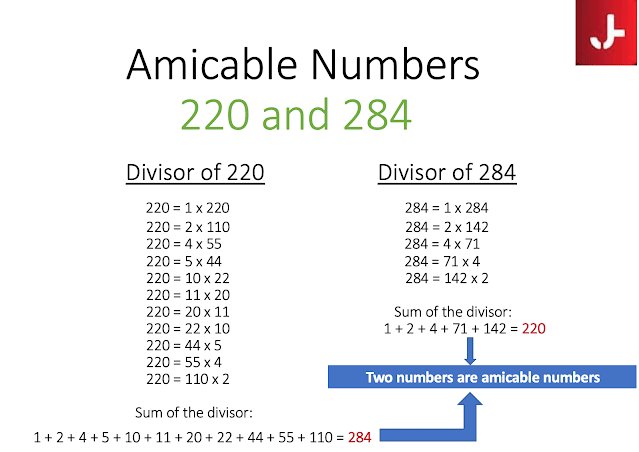# Amicable number in Java with examples [2 ways]

In this post, I will be sharing what is an amicable number in Java, examples of amicable numbers, algorithms, and programs to check whether a given number is an amicable number or not. It is also known as friendly numbers.

First, we need to understand the term proper divisor. A number is called a proper divisor if a number is a divisor other than the number itself.

## What is an Amicable Number

A pair of numbers is said to be amicable numbers if
1. the sum of the proper divisors of each(excluding the number itself) is equal to the other number.
2. they are different.

Examples :

1. 220 and 284 are amicable numbers as shown below in the image2. 1184 and 1210 are amicable numbers

## Java Program for Amicable Numbers

### Method1: Using for loop

```import java.util.Scanner;
public class AmicableNumber {
public static void main(String args[]) {
Scanner scan = new Scanner(System.in);
System.out.println("First Number: ");
int num1 = scan.nextInt();
System.out.println("Second Number: ");
int num2 = scan.nextInt();
int firstDivisorSum = 0, secondDivisorSum = 0;
for( int i=1; i < num1 ; i++)
{
if(num1 % i == 0)
firstDivisorSum += i;
}
for( int i=1; i < num2 ; i++)
{
if(num2 % i == 0)
secondDivisorSum += i;
}
if((num1 == secondDivisorSum) && (num2 == firstDivisorSum))
{
System.out.println(num1 + " and " + num2 + " are amicable numbers in Java");
}
else
{
System.out.println("Given numbers are NOT amicable numbers");
}
}
}
```

Output:

First Number: 220
Second Number: 284
220 and 284 are amicable numbers in Java

### Method 2:

```import java.util.Scanner;

public class AmicableNumber2 {
public static void main(String args[]) {
Scanner scan = new Scanner(System.in);
System.out.println("First Number: ");
int num1 = scan.nextInt();
System.out.println("Second Number: ");
int num2 = scan.nextInt();
int sum1 = 0, sum2 = 0;
for( int i=1; i <= num1 ; i++)
{
if(num1 % i == 0)
sum1 += i;
}
for( int i=1; i <= num2 ; i++)
{
if(num2 % i == 0)
sum2 += i;
}
if(sum1 == sum2)
{
System.out.println(num1 + " and " + num2 + " are amicable numbers in Java");
}
else
{
System.out.println("Given numbers are NOT amicable numbers");
}
}
}
```

Output:

First Number: 1184
Second Number: 1210
1184 and 1210 are amicable numbers in Java

## Algorithm: Steps to find an Amicable number

1. Enter the two numbers to check for amicable numbers.

2. Find all the divisors of both numbers.

3. Find the sum of the divisors of both numbers.

4. Check if the sum of divisors of one number is equal to the other input number or not.

That's all for today. Please mention in the comments if you have any questions related to how to check that given numbers are amicable numbers in Java with examples.